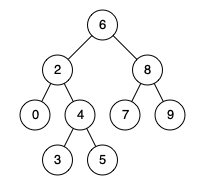0235. Lowest Common Ancestor of a Binary Search Tree

# 235. Lowest Common Ancestor of a Binary Search Tree#

## 题目 #

Given a binary search tree (BST), find the lowest common ancestor (LCA) of two given nodes in the BST.

According to the  definition of LCA on Wikipedia: “The lowest common ancestor is defined between two nodes p and q as the lowest node in T that has both p and q as descendants (where we allow a node to be a descendant of itself).”

Given binary search tree: root = [6,2,8,0,4,7,9,null,null,3,5]Example 1:

``````Input: root = [6,2,8,0,4,7,9,null,null,3,5], p = 2, q = 8
Output: 6
Explanation: The LCA of nodes 2 and 8 is 6.
``````

Example 2:

``````Input: root = [6,2,8,0,4,7,9,null,null,3,5], p = 2, q = 4
Output: 2
Explanation: The LCA of nodes 2 and 4 is 2, since a node can be a descendant of itself according to the LCA definition.
``````

Note:

• All of the nodes’ values will be unique.
• p and q are different and both values will exist in the BST.

## 解题思路 #

• 在二叉搜索树中求两个节点的最近公共祖先，由于二叉搜索树的特殊性质，所以找任意两个节点的最近公共祖先非常简单。

## 代码 #

``````
package leetcode

/**
* Definition for TreeNode.
* type TreeNode struct {
*     Val int
*     Left *ListNode
*     Right *ListNode
* }
*/
func lowestCommonAncestor(root, p, q *TreeNode) *TreeNode {
if p == nil || q == nil || root == nil {
return nil
}
if p.Val < root.Val && q.Val < root.Val {
return lowestCommonAncestor(root.Left, p, q)
}
if p.Val > root.Val && q.Val > root.Val {
return lowestCommonAncestor(root.Right, p, q)
}
return root
}

``````